# 通过仿真解释“音叉之谜”

2018年 4月 13日

### 音叉之谜

Standupmaths 在 YouTube 上发布的一条视频中，科学爱好者 Matt Parker 和 Hugh Hunt 讨论并演示了关于音叉的“奥秘”：当敲打音叉并将其放在桌面上时，发出声音的频率似乎加倍了。事实上，这个谜团可以用非线性固体力学来解释。

### 声音如何到达我们的耳朵？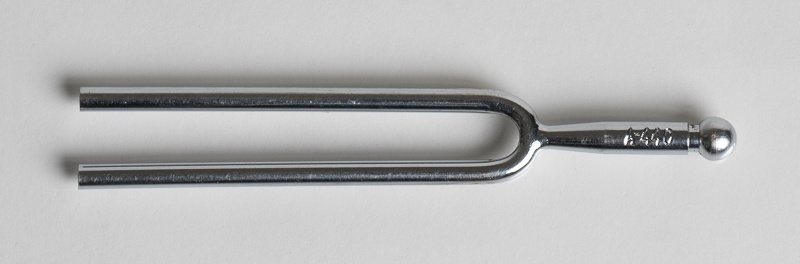1. 音叉的弯曲伴随着在音叉横截面上线性变化的上下运动
2. 如牛顿第二定律所要求的，柄具有基本刚性的轴向运动，这对于将质心保持在固定位置是必要的。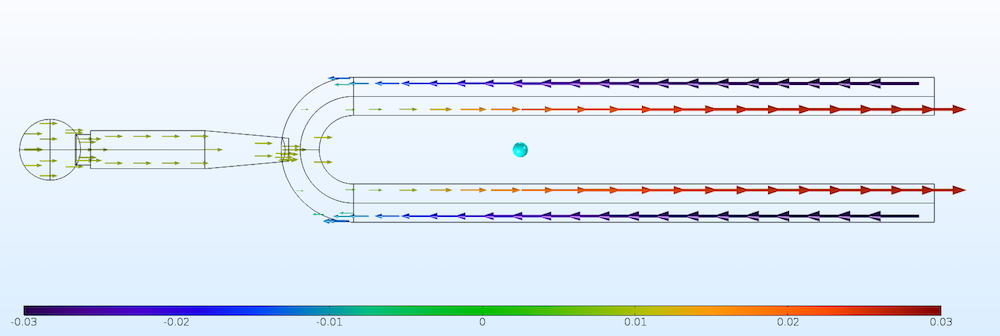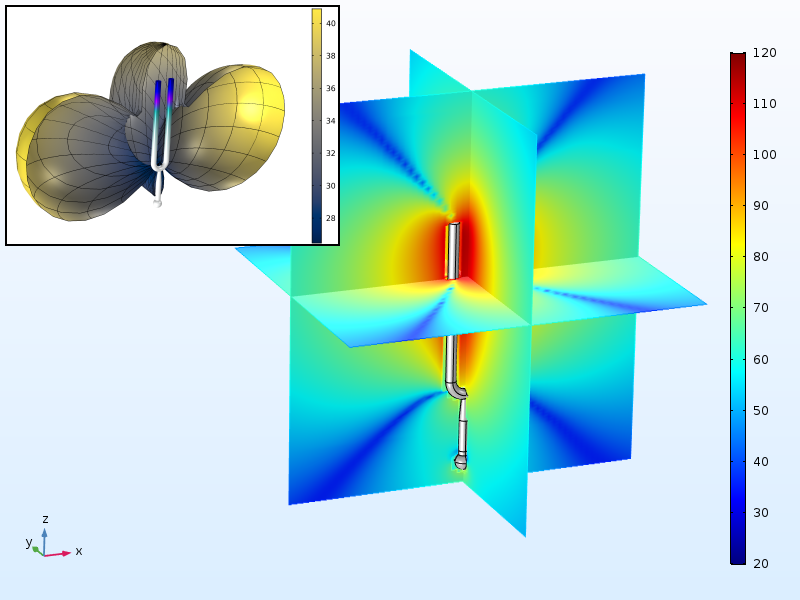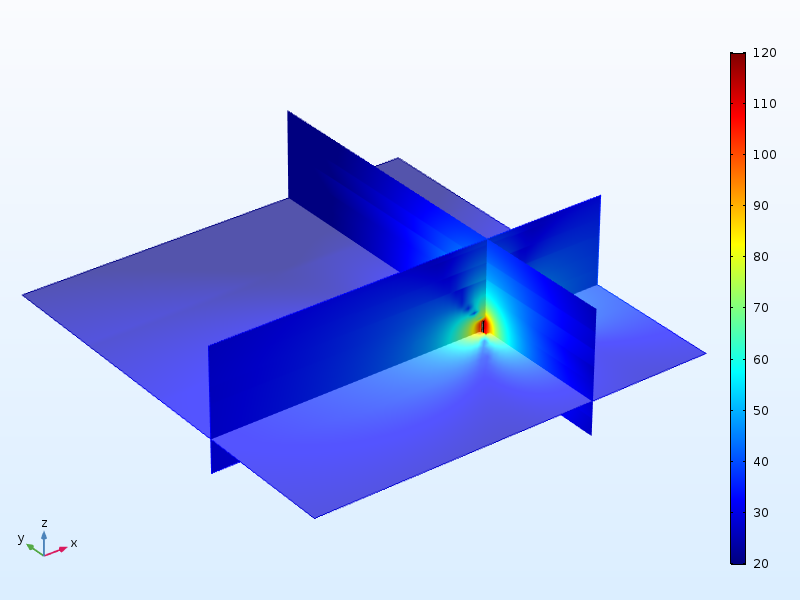### 倍频是固有频率吗？

f_1 = \dfrac{1.875^2}{2 \pi L^2}\sqrt{\dfrac{EI}{\rho A}}

• 音叉长度，L
• 杨氏模量，E；钢通常约为 200GPa
• 质量密度，ρ；约 7800kg/m 3
• 音叉横截面的面积惯性矩，I
• 音叉的横截面积，A

f_2 = \dfrac{4.694^2}{2 \pi L^2}\sqrt{\dfrac{EI}{\rho A}}

### 音叉之谜的可能原因

\dfrac{\delta Z}{L} = \beta \left ( \dfrac{a}{L} \right)^2

\delta Z = 0.2 \left ( \frac{1}{ 80} \right)^2 \mathrm 80 \, mm = 0.0025 \, mm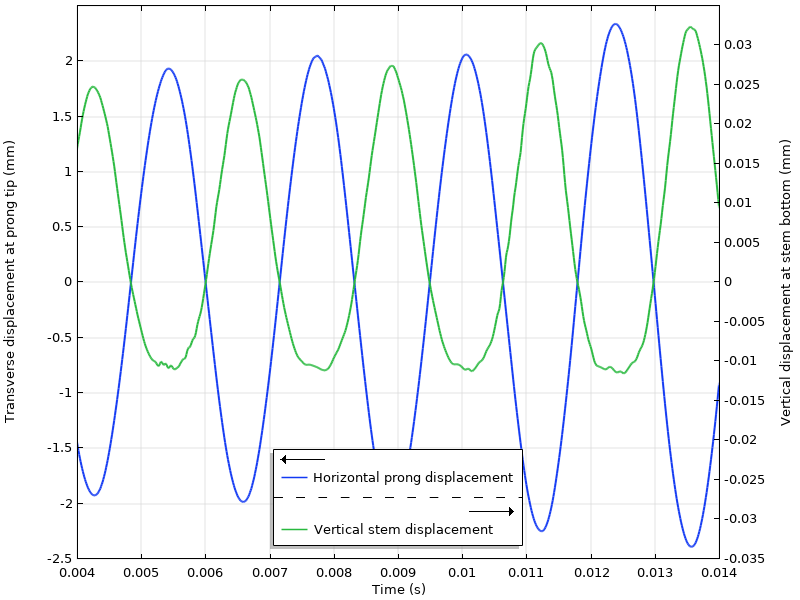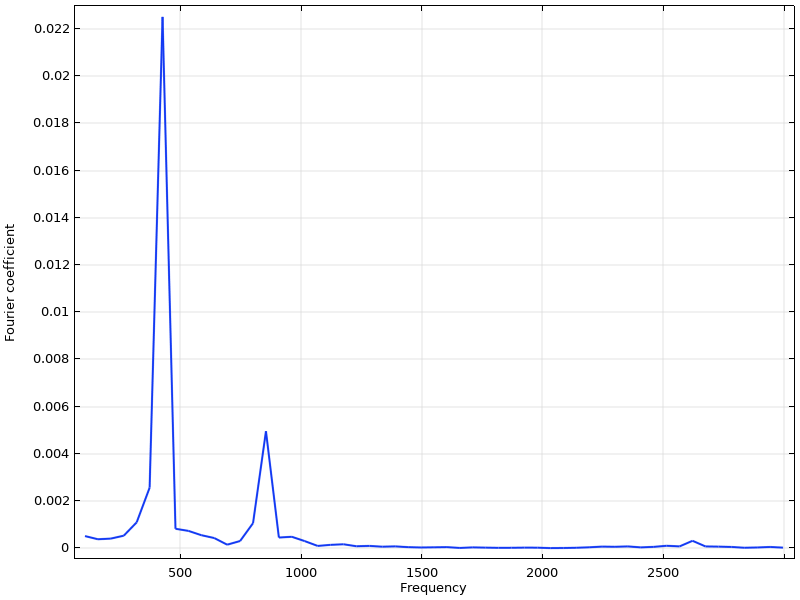### 不同的音叉

standupmaths 的原始视频的评论者已经注意到，某些音叉的效果比其他音叉好，而对于某些音叉，很难看到频率加倍。如上所述，第一个标准是您要用力敲击以进入非线性状态。但是，也存在影响两种振动幅度之间比率的几何差异。

f_1 = \dfrac{1.875^2} {2 \pi L^2}\sqrt{\dfrac{EI}{\rho A}}

I = \dfrac{d^4} {12}

I = \dfrac{\pi d^4} {64}

### 后续步骤

• 在 YouTube 上观看 standupmaths 的原始视频：
• 在 COMSOL 博客上了解有关音叉和仿真的交集的更多信息：